## Indicators

•Cited by SciELO
•Access statistics

•Cited by Google
•Similars in SciELO
•Similars in Google

## Print version ISSN 0122-3461On-line version ISSN 2145-9371

### Ing. Desarro.  no.24 Barranquilla July/Dec. 2008

Controling a planar temperature grid

Andrés Julián Saavedra-Montes*, Liliana Fernández-Samacá**

*Electrical Engineer, Master in Systems of Electrical Energy Generation and PhD student in Engineering, Universidad del Valle. Assistant professor in the School of Electrical and Mechanical Engineering at Universidad Nacional, Medellin.ajsaaved@unal.edu.co Address: Universidad del Valle, Ciudad Universitaria Meléndez, Edificio 353 Oficina 20-35. Cali, (Colombia). Telephone: (572)3391780- Ext 111.

** Electronics Engineer, Universidad Pedagógica y Tecnológica de Colombia. Master in Industrial Automation, Universidad Nacional, Manizales. PhD student in Engineering at Universidad del Valle. Assistant professor in Universidad Pedagógica y Tecnológica de Colombia. lifersa@univalle.edu.co

Grants and support: Saavedra Montes, A.J. acknowledges a fellowship for doctoral student from Colciencias (Instituto Colombiano para el Desarrollo de la Ciencia y la Tecnología Francisco José de Caldas), and a study commission from Universidad Nacional de Colombia. Fernández-Samacá, L. akcnowledges a study commisssion from Universidad Pedagógica y Tecnológica de Colombia.

Fecha de recepción: 22 de febrero de 2008
Fecha de aceptación: 30 de abril de 2008

Resumen

Este artículo presenta el diseño de controladores multivariables, uno distribuido y otro centralizado, para una rejilla plana de temperatura. El objetivo es mantener una superficie de temperatura constante en la rejilla. Aquí, presentamos el modelado del sistema a partir de pruebas escalón. También, presentamos el modelado del sistema con incertidumbres paramétricas en las constantes de tiempo. Para analizar el modelo del sistema, utilizamos la descomposición de valores singulares y el arreglo de ganancias relativas. Dos estrategias de control son aplicadas a el sistema, un controlador H y un controlador multivariable PID. La rejilla de temperatura plana es útil para mostrar un sistema mal condicionado, el cual necesita análisis complejo.

Palabras claves: Modelo de incertidumbres, control robusto, sistema mal condicionado, controladores multivariables.

Abstract

This paper presents the design of multivariable controllers, one distributed and another centralized, for a planar temperature grid. The objective is to keep a constant temperature surface on the grid. Herein, we present the modeling of the system by using step tests. Also, we present the modeling of the system with parametric uncertainties in time constants. To analyze the system model, we use the singular values decomposition and the relative gain array. Two control strategies are applied to the system, an controller and a multivariable PID. The planar temperature grid is useful for showing an ill-conditioned system, which needs complex analysis.

Key words: Uncertainty model, robust control, ill-conditioned system, multivariable controllers.

INTRODUCTION

A planar temperature grid is an example of an ill-conditioned multivariable system useful for analyzing and designing multivariable controllers. This system is affected by disturbances like: environment temperature and wind currents, inter-zone effects, and self-induced wind currents .

The planar temperature grid had been used as a study case in resource allocation. Many works have applied bio-inspired solutions to achieve uniform temperature on the grid. In  a Honey Bee social foraging algorithm is used. An algorithm based on the Bacteria Swarm foraging is presented in . An Ant Colony system is also used, for example the work presented in  . Two papers show how allocation strategy can achieve an Ideal Free Distribution (IFD) to achieve the maximum uniform temperature on grid under multivariable saturation constrains  .  presents how the foraging theory is applied to track a desired temperature. In general, these works apply intelligent control techniques to obtain uniform temperature on a planar temperature grid. Herein, we propose another way to control this system.

The objective of this paper is to present the modeling of the temperature grid by means of a transfer matrix to design controllers via modern control theory. The control theory has tools to analyze the coupling level between inputs and outputs, and indexes to calculate the robustness and the stability of the system; therefore, we chose the modern control theory to model and control the planar temperature grid.

We propose two ways to model a system by using symmetrical positions of the actuators and temperature zones. To analyze the system we use singular value decomposition (SVD), relative gain array (RGA), and optimal condition number (OCN). Also, we present two controllers, one centralized and another distributed, for an uncertainty model of a system.

This paper is organized as follows: In the system modeling section, we present the selection, identification, validation, and analysis of the model.

In the controller design section, we present the grid performance with H controller and a multivariable PID. Finally, we present the conclusions, acknowledgment, and references.

SYSTEM MODELING

In this work, we used a platform composed of a planar temperature grid and a data acquisition system. The planar temperature grid has 16 light bulbs, actuators, and 25 LM35 integrated-circuit temperature sensors. The grid is shown in Figure 1. The data acquisition system has signal conditioners connected to a computer through its serial port, and a man-machine interface called MuTEPCO designed with GUI from Matlabtmhe interface to monitor the sensor signals, drive the actuators, show the data in screen, and define control algorithms. The detailed description of the platform appears in .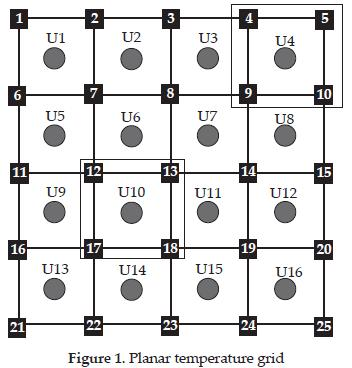This platform belongs to the research group Perception and Intelligent System (PSI) research group at Universidad del Valle in Colombia. The PSI built this platform to emulate complex processes, e.g. ill-conditioned multivariable systems, and the PSI uses the platform to design and test resource allocation algorithms.

In the next sub-sections, we present two ways to model the planar temperature grid with transfer functions. Firstly, we propose a method to model the system based on the location of sensors and actuators. Then, we model the system observing the temperature profile on the planar grid.

1.1. Modeling by symmetrical positions

To obtain squared transfer matrix, we defined actuator signals as inputs and the temperature average per cell as outputs. Each cell is conformed by one actuator and the four sensors around it; see Figure 1.

Each output is defined by (1).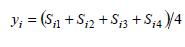Where yi is the i-th measured average temperature corresponding to the four sensors (sij with j=1, 2, 3, and 4) of the i-th cell for i-th input. The inputs are pulse width modulation signals (PWM) and they drive the actuators. The excursion of each input signal is from 0 to 1 and corresponds to pulse width. The system has 16 inputs and 16 outputs; therefore, the multivariable system model is a 16 × 16 transfer matrix that consists of 256 transfer functions.

The structure of the planar temperature grid allows deducting all transfer functions obtaining only 36 transfer functions that can be found via three step-signal tests. For example, note that the location of cell 1 is similar to location of cell 4; both have the same number of cells around them. Then, if all actuators present similar dynamics, the transfer function of cell 1 with respect to cell 2 (Y1/U2) is similar to the transfer function between cell 4 and cell 8 (Y4/U8), and to the transfer function between the cell 13 and cell 9 (Y13/U9). Three experiments to find the 36 transfer function could be made; these experiments are described as follows:

In the first experiment, it is necessary to apply a step signal in u1 and to measure the y1, y2, y3, and y4 outputs; y5, y9, and y13 outputs did not require to be measured because their locations with respect to u1 are similar to locations of y2, y3, and y4 outputs; hence, the transfer functions of y5, y9, and y13 with respect to u1 are equal to transfer functions of y2, y3, and y4 with respect to u1. Next, y7, y8, and y12 outputs must be measured and y10, y14, and y15 can be found by symmetry. Finally, each output on the grid diagonal must be measured separately. The dynamics obtained with respect to u1 are similar to dynamics with respect to u4, u13, and u16 inputs, e. g., the Y4/U1 transfer function is equal to the Y1/U4 transfer function.

In the second experiment, a step signal is applied in u8 and each grid output must be measured separately. The sixteen outputs must be registered via this experiment. The dynamics to u3, u2, u9, u14, u15, and u12 inputs can be obtained by symmetry, because the locations of these inputs are symmetric to the location of the u8.

In the third experiment, a step signal is applied in u10 and the y10, y9, y5, and y1 outputs must be measured; y14, y15 and y16 can be obtained by symmetry. The y11, y8, and y12 outputs also require be measured and y6, y2, and y3, outputs can be found by symmetry. Finally, each y13, y7, and y4 output is measured separately. The dynamics for u10 are similar for the inputs u11, u6 and u7; e.g., the transfer function Y7/U10 is equal to the transfer function Y10/U7.

The previous modeling can be used as long as the dynamic characteristics of actuators are similar, otherwise, each transfer function must be obtained separately. We ran tests over actuators and noted that the dynamic characteristics of actuators are different; hence, it is not possible to use modeling by symmetrical locations. Furthermore, to calculate the overall model, getting each transfer function separately, long experimental time is necessary because each step test requires 20 minutes.

1.2. Modeling by temperature zones

We ran open-loop tests for different PWM percentages and actuator signals, and we observed that temperature surface conserves a distribution by regions, independent of its average temperature value. We obtained the planar temperature grid model by using two temperature zones, see Figure 2. The temperature averages on each zone are the system outputs. Therefore, the system has two outputs. The inputs are PWM percentages applied over actuators in each temperature zone. The system model was reduced from a transfer matrix with 256 elements to a transfer matrix with four elements.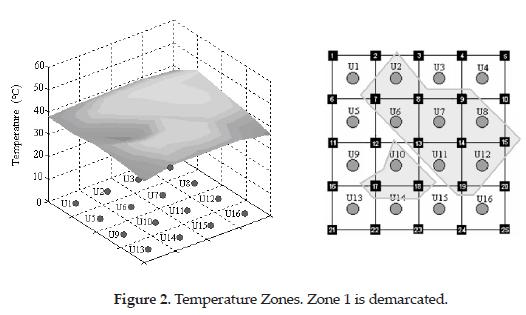The u2, u7, u6, u8, u11, u12, and u10 actuators belong to zone 1 and the others to zone 2. Sensors 7, 8, 9, 13, 14, 15, 19, 18, and 17 (Figure 1) measure the temperature in zone 1; the remaining sensors measure the temperature in zone 2. Zone 1 has 9 sensors and 7 actuators, and zone 2 has 16 sensors and 9 actuators. To obtain the system model we applied step signal in the inputs, and measured the average temperature per zone, system outputs. The step test allows defining the order of the transfer function of system model.

1.2.1. Selection, identification, and validation of the model

We selected a first order model for each transfer function of the transfer matrix. The transfer matrix has four components, one per input-output combination. Each block has a gain and a time constant. Figure 3 shows the links between inputs and outputs.

We saw the effect of each input over each output separately and we obtained the transfer functions observing the natural response curves of the system, seeFigure 4. Transient analysis of the step test was used to estimate parameters of the transfer functions that relate each input to each output. To identify the system model, this was considered as linear plant; therefore, each plant output is the sum of outputs due to each input, see Figure 3.A step signal was applied to each input and each output signal was recorded. The parameters for each block were calculated from the curves obtained by using 'ident' from MATLABTM. Before the identification of the model, the curves were adjusted with the curve-fitting tool from MATLABTM because these had much noise. Figure 4 shows the Y1 and Y2 outputs for the step applied in the U2 input. The step signal was applied in steady state. The delay was not taken into account, because the time constant is large. The four transfer functions obtained are presented in Table 1. The scaling transfer matrix was calculated with maximum temperature achieved during the experiment, 61.5° C.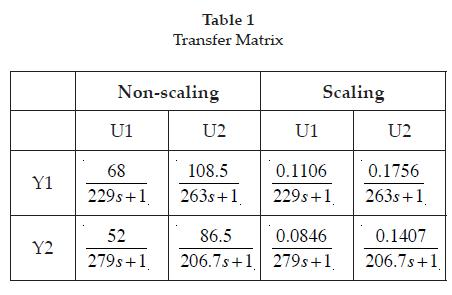To validate the model, we compared model responses to the responses of the real system. Figure 4 shows system and model response by step signal applied on U2 input.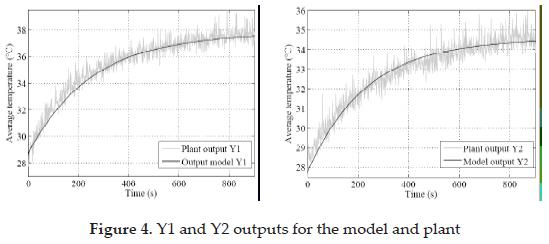1.3. Uncertainty Modeling

To design controllers, it is necessary to obtain a model of a system coherent with the control objective. In practice, the model is different from the real system for many reasons, i.e., system parameters change, there are mistakes in sensor measurements, or the model does not include all dynamics (nonmodeled dynamics). Some differences between the system modeled and the real system can be included in an uncertainty model. Therefore, to design controllers the use of models with uncertainties is recommended. We defined that said system has parametric multiplicative uncertainties (2) over time constants.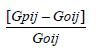Initially, we obtained a model per input-output pair, see Table 2, but we observed that uncertainty models are similar; then, we decided to find a general uncertainty model (structured multiplicative uncertainty model), based on the result presented by .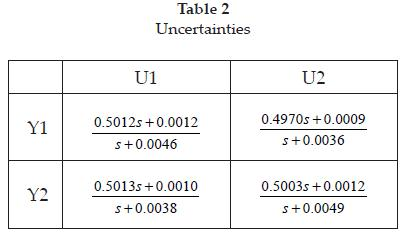This model is obtained with minimum singular value of relative error of σ[Go -1[Gp -Go]], varying the parameters of interest, in this instance the time constants of transfer functions. The uncertainty function is defined by (3).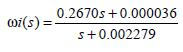We obtained the frequency response of minimum singular value of the system through variation of 20% on time constants. We defined a weight function that covers the sweep of frequency response or the uncertainty region for minimum singular value, see Figure 5.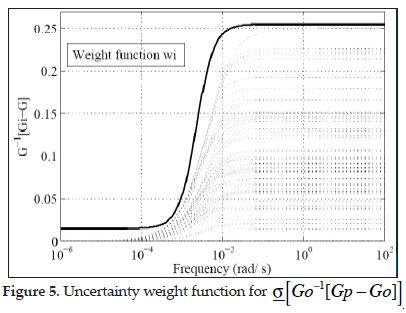1.4. System analysis

To analyze the system, we used the condition number, CN, the optimal condition number, OCN, and the relative gain array, RGA. These indexes were obtained by the plant model.

The steady state transfer matrix was decomposed in singular values, the minimum singular value is σ = 0.0027 and the maximum singular value is σ = 0.2646 . The condition number is CN = σ/σ = 99.2181. This value indicates that the system is ill-conditioned and presents sensitivity among outputs and inputs.

The input-output pairs were determined from the relative gain array. These pairs were used to design the decentralized feedback control. The relative gain array (Λ) was obtained for two frequencies ω = 0 and ω = ∞. The relative gain arrays analyzed are in (4) and (5).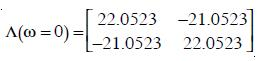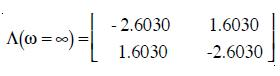Based on useful properties of the relative gain array Λ(ω=0) to control, the input-output pairs corresponding to positive values of relative gain array should be selected; therefore, we selected Y1/U1 and Y2/U2.

The condition number is also calculated. This was obtained from model for scaling plant. The minimized or optimal condition number is a more reliable factor. This number was calculated from the relative gain array on steady state. The sum-norm of the relative gain array in ω = 0 calculates the optimal condition number (6).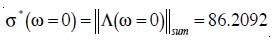By comparing the optimal condition number to the condition number obtained from singular values, we observed that these are closed and concluded that the system is ill-conditioned.

2. controller design

Herein, we present two multivariable controllers, one centralized controller and another distributed controller. The design of the two controllers is based on the analysis of the system. To design the controllers, we used a weight function that includes the requirements for each controller. Performance and stability of the plant with each controller were evaluated by using the µ analysis. To validate the controllers, we present the simulation results for one tracking test and one regulation test.

2.1. Decentralized controller design

We selected a K-diagonal controller, which is a decentralized controller. To design the controller, we used relative gains array (RGA) in w = 0. We chose input-output pairs for non-negative elements of the RGA from (4), . The input-output pairs chosen are Y1/U1 and Y2/U2, obtaining a diagonal transfer matrix.

We made an experimental design of PID controllers for input-output pair and took into account the uncertainty models. The requirements for the controller design decrease the system's stabilization time and allow acceptable overshoot. We defined the new time constant as half of system time constant in open loop. We chose the minimum time constant, the transfer matrix, to define the new time constant (τij = 0.8 τij). We used a damper factor ρ = 0.7. This damper factor establishes an overshoot under 25%. The controller transfer functions calculated are presented below:

k11 controller function for Y1/U1 is: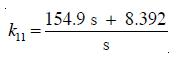k22 controller function for Y2/U2 is: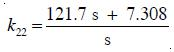To evaluate the performance of the controllers, we chose a weight for the sensitivity function (S). The sensitivity function is a good indicator of close-loop performance for multivariable systems. The weight chosen is represented by (9):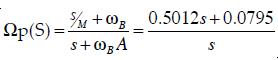Where, ωB = 0.0795 is the minimum natural frequency between Y1/U1 and Y2/U2 transfer functions. We selected the sensitivity function magnitude M=1.9953 for all outputs, and maximum steady state error A= 0 to ensure integral action. To complement the performance evaluation, we also used the µ analysis.

Figure 6a shows two weight functions and the sensitivity function of system with K-diagonal controller. The weight function with ωB = 0.0795 is less than the sensitivity function at some frequencies. This weight function is restrictive for the system; hence, we chose a less restrictive minimum natural frequency ωB = 0.001. See Figure 6a.

Figure 6b shows the nominal performance of the K controller by µ analysis. For the weight function with ωB = 0.0795, the controller does not have good performance since the nominal performance is greater than one in most frequencies. With a less restrictive weight function, e.g., using ωB = 0.001, nominal performance improves.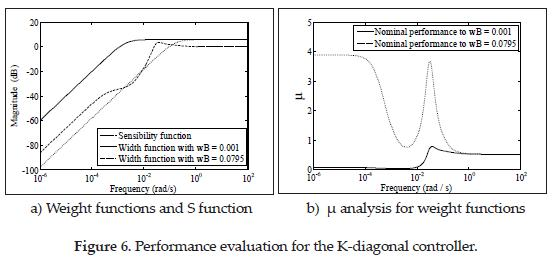For ωB = 0.0795, robust performance and robust stability of the system are shown in Figure 7. The nominal and robust performances are greater than one for most frequencies; however, the robust stability is less than one, i.e. the system presents robust stability for the full range of frequencies.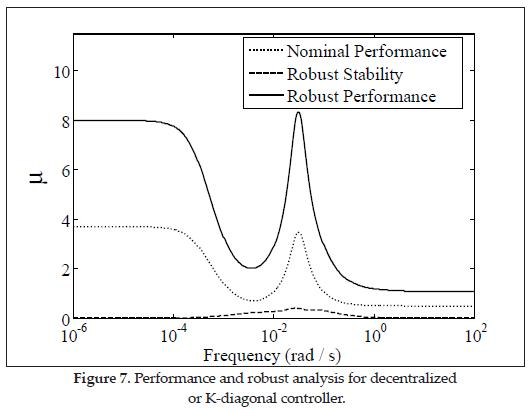The controllers were tested separately for each input-output pair and achieved desired specifications. When we tested the controlled system, the stabilized time was less than the specified time. The controllers have good regulation and track the reference correctly. See Figure 8.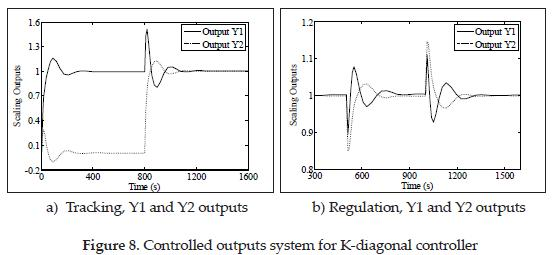2.2. Design of robust H∞ controller

The other control strategy chosen was an H control. A generalized plant model P was obtained and a controller was designed from the algorithms proposed in . The generalized plant order is 6 inputs, 6 outputs, and 8 states. The H∞ controller dynamic has 2 outputs, 2 inputs, and 2 states.

To evaluate the performance of the controllers, we used the µ analysis.Figure 9 shows the robust stability, robust performance, and nominal performance of the system with an H∞ control. Performance evaluation was made for ωB = 0.0795 and wB = 0.001.

Figure 9a shows that the robust and nominal performances are greater than one at low frequencies, which is bad performance at these frequencies. For ωB = 0.001, the nominal performance has been improved, but robust performance remains grater than one. See Figure 9b.

figure 9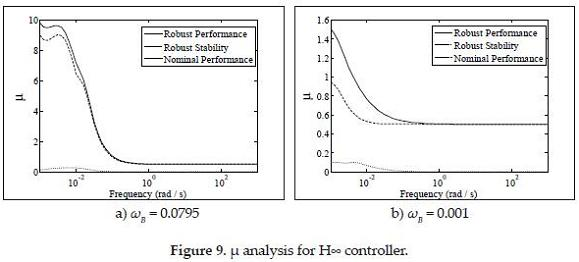The H∞ controller responses for the tracking and regulation tests are shown in Figure 10 In the regulation test, the temperature grid model with H∞ controller presents an overshot of about 12%. In the same test, the model with the K-diagonal controller presents an overshot of about 16%. In the regulation test, the grid model with the H controller presents a stabilization time of 200s. See Figure 10b. This time is less than the stabilization time presented by the model with the K-diagonal controller, which was approximately 300s.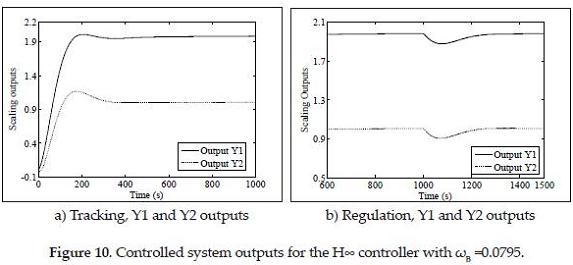3. CONCLUSION

An ill-conditioned multivariable system was identified by using transient analysis of step response. We chose a model based on temperature distribution, obtained matrix transfer parameters through experimental tests, and validated the model by comparing real response to simulated response. The model considers parametric uncertainties over time constants.

The system was analyzed from singular value decomposition and relative gain array. We calculated the condition number and optimal condition number, which showed that the system is ill-conditioned. The frequency response analysis of RGA elements allowed selection input-output pair to design PI decentralized controllers.

When the temperature grid was represented with a model in the Laplace domain, then, we could apply the analysis tools to evaluate the robust stability and the nominal and robust performance, we used the µ analysis. We compared the decentralized control strategy with H∞ centralized control strategy through µ analysis and controller response to track and regulate the nominal model. This comparison shows a compromise between response speed and robust performance and stability.

The measured signals have noise; this makes it difficult to identify the model. Other identification techniques can be used to obtain more accurate models. To improve the model presented in this paper, more temperature zones can be defined, but this implies an increase in the order of the transfer matrix of the system and complexity of its analysis.

Acknowledgment

Authors are grateful to the engineers Wilfredo Alonso Morales and Javier Castillo for his important collaboration in the development of experimental tests.

REFERENCES

 QUIJANO, N., GIL, A. E. & PASSINO, K. M. (2005) Experiments for dynamic resource allocation, scheduling, and control: new challenges from information technologyenabled feedback control. Control Systems Magazine, IEEE, 25, 63-79.        [ Links ]

 QUIJANO, N. & PASSINO, K. M. (2007) Honey Bee Social Foraging Algorithms for Resource Allocation, Part II: Application. IN PASSINO, K. M. (Ed.) American Control Conference, 2007. ACC '07.        [ Links ]

 MUÑOZ, M. A., LÓPEZ, J. A. & CAICEDO, E. (2007) Bacteria Swarm Foraging Optimization for Dynamical Resource Allocation in a Multizone Temperature Experimentation Platform. Analysis and Design of Intelligent Systems using Soft Computing Techniques. Springer.        [ Links ]

 MUÑOZ, M. A.,LÓPEZ , J. A. & CAICEDO, E. F. (2007) Ant Colony Optimization for Dynamical Resource Allocation in a Multizone Temperature Experimentation Platform. Latin America Transactions, IEEE (Revista IEEE America Latina), 5, 81-86.        [ Links ]

 MUÑOZ, M., A.,LÓPEZ , J., A. & CAICEDO, E. (2006) Ant Colony Optimization for Dynamical Resource Allocation in a Multizone Temperature Experimentation Platform. IN JESUS, A. L. (Ed.) Electronics, Robotics and Automotive Mechanics Conference, 2006.        [ Links ]

 QUIJANO, N. & PASSINO M., K. (2007) The Ideal Free Distribution: Theory and Engineering Application. Systems, Man, and Cybernetics, Part B, IEEE Transactions on, 37, 154-165.        [ Links ]

 QUIJANO , N. & PASSINO , K. M. (2006) Optimality and stability of the ideal free distribution with application to temperature control. IN PASSINO, K. M. (Ed.) American Control Conference, 2006.        [ Links ]

 QUIJANO , N.,PASSINO , K. M. & ANDREWS, B. W. (2006) Foraging theory for multizone temperature control. Computational Intelligence Magazine, IEEE, 1, 18-27.        [ Links ]

 MUÑOZ , M. A.,LÓPEZ , J. A. & CAICEDO , E. F. (2005) Implementation of a distributed control experimentation platform. International Conference on, Industrial Electronics and Control Applications, 2005. ICIECA 2005.        [ Links ]

 VADIGEPALLI, R., GATZKE, E. P. & DOYLE, F. J. (2001) Robust Control of a Multivariable Experimental Four-Tank System.        [ Links ]

 SKOGESTAD, S. & POSTLETHWAITE, I. (1996) Multivariable feedback control: analysis and design.        [ Links ]All the contents of this journal, except where otherwise noted, is licensed under a Creative Commons Attribution License